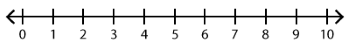##### Notes

Daily Math Practice provides a week's worth of problems ideal for morning work or review. Week 21 reviews standards from previous weeks and supports Common Core Mathematics Standards K.OA.A.1, K.OA.A.2, & K.OA.A.3.

##### Print Instructions

NOTE: Only your test content will print.
To preview this test, click on the File menu and select Print Preview.

See our guide on How To Change Browser Print Settings to customize headers and footers before printing.

# Kindergarten - Daily Math Practice - Week 21 (Kindergarten)

Print Test (Only the test content will print)
 DAILY MATH PRACTICE - WEEK 21 DAY 1 DAY 2 Subtract. Use the number line.7 - 4 = Subtract. Use the number line.5 - 4 = Grant has 3 thank you notes to write. He wrote 1 note so far. How many thank you notes does Grant have left to write? Draw a picture:Answer:thank you notes Mike has 9 raffle tickets to sell. He sells 5 of the tickets to his family. How many raffle tickets does Mike have left to sell?Write a subtraction sentence:Answer:raffle tickets Circle the addition sentence for the model.5 + 4 = 96 + 3 = 9 Circle the addition sentence for the model.2 + 7 = 91 + 8 = 9 Count by tens. Circle the number that comes next.10, 20, 30, 40, 50, ?90 or 60 Draw a square using 2 triangles.

 DAILY MATH PRACTICE - WEEK 21 DAY 3 DAY 4 Subtract. Use the number line.6 - 5 = Subtract. Use the number line.4 - 2 = Jess has 2 softball gloves. She gives 1 glove to her sister. How many softball gloves does Jess have left?Draw a picture:Answer:softball gloves There are 10 stickers on a sheet. Ms. Klein uses 8 of the stickers. How many stickers are left on the sheet?Write a subtraction sentence:Answer:stickers Circle the addition sentence for the model.5 + 4 = 93 + 6 = 9 Circle the addition sentence for the model.7 + 2 = 91 + 8 = 9 Count forward. Circle the number that comes next.73, 74, 75, ?78 or 76 Write the missing number.13 = 1 ten +onesYou need to be a HelpTeaching.com member to access free printables.